### The State Space Model

The state space model represents a multivariate time series through auxiliary variables, some of which might not be directly observable. These auxiliary variables are called the state vector. The state vector summarizes all the information from the present and past values of the time series that is relevant to the prediction of future values of the series. The observed time series are expressed as linear combinations of the state variables. The state space model is also called a Markovian representation, or a canonical representation, of a multivariate time series process. The state space approach to modeling a multivariate stationary time series is summarized in Akaike (1976).

The state space form encompasses a very rich class of models. Any Gaussian multivariate stationary time series can be written in a state space form, provided that the dimension of the predictor space is finite. In particular, any autoregressive moving average (ARMA) process has a state space representation and, conversely, any state space process can be expressed in an ARMA form (Akaike 1974). More details on the relation of the state space and ARMA forms are given in the section Relation of ARMA and State Space Forms.

Let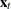be the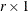vector of observed variables, after differencing (if differencing is specified) and subtracting the sample mean. Let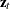be the state vector of dimension s,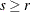, where the first r components ofconsist of. Let the notation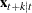represent the conditional expectation (or prediction) of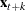based on the information available at time t. Then the lastelements ofconsist of elements of x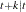, where k >0 is specified or determined automatically by the procedure.

There are various forms of the state space model in use. The form of the state space model used by the STATESPACE procedure is based on Akaike (1976). The model is defined by the following state transition equation :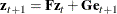In the state transition equation, thecoefficient matrix F is called the transition matrix; it determines the dynamic properties of the model.

Thecoefficient matrix G is called the input matrix; it determines the variance structure of the transition equation. For model identification, the first r rows and columns of G are set to anidentity matrix.

The input vector eis a sequence of independent normally distributed random vectors of dimension r with mean 0 and covariance matrix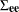. The random error eis sometimes called the innovation vector or shock vector.

In addition to the state transition equation, state space models usually include a measurement equation or observation equation that gives the observed valuesas a function of the state vector. However, since PROC STATESPACE always includes the observed valuesin the state vector, the measurement equation in this case merely represents the extraction of the first r components of the state vector.

The measurement equation used by the STATESPACE procedure is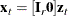where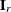is anidentity matrix. In practice, PROC STATESPACE performs the extraction offromwithout reference to an explicit measurement equation.

In summary:

xis an observation vector of dimension r.

zis a state vector of dimension s, whose first r elements are xand whose lastelements are conditional prediction of future x.

F

is antransition matrix.

G

is aninput matrix, with the identity matrix Iforming the first r rows and columns.

eis a sequence of independent normally distributed random vectors of dimension r with mean 0 and covariance matrix.